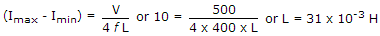# Electronics and Communication Engineering - Power Electronics

### Exercise :: Power Electronics - Section 3

26.

To protect an SCR against high di/dt we connect

 A. a capacitance in series with SCR B. a capacitance in parallel with SCR C. an inductance in parallel with SCR D. an inductance in series with SCR

Explanation:

current through inductance cannot change instantaneously.

27.

In a parallel inverter

 A. the commutating capacitor C is across the primary of transformer and the load is across the secondary B. the commutating capacitor and load are connected across the secondary of transformer C. the commutating capacitor C and load are connected in series and the combination is across the secondary of transformer D. none of the above

Explanation:

No answer description available for this question. Let us discuss.

28.

A silicon controlled switch has :

Explanation:

Anode, cathode, anode gate and cathode gate.

29.

A dc chopper V = 500 V feeds an electric fraction dc series motor. The chopping frequency is 400 Hz. The series inductance required to limit the maximum current swing to 10 A is

 A. 20 mH B. about 31 mH C. about 51 mH D. about 92 mH

Explanation:.

30.

A single phase fully controlled bridge rectifier is fed by a source having inductance. The output voltage during overlap period is

 A. Vm B. 0.5 Vm C. zero D. 0.5 Vm - voltage drop across inductance